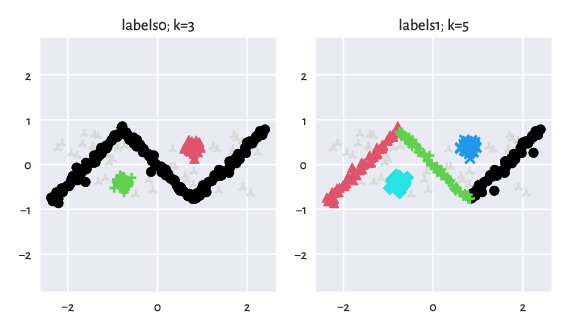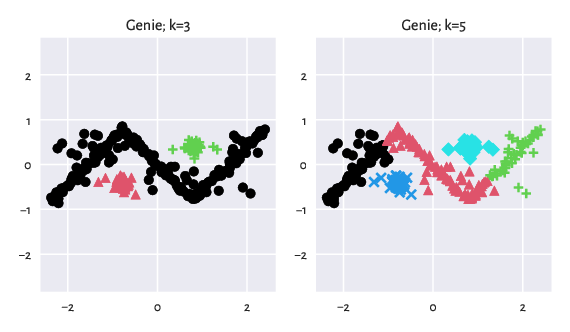# There Can Be Many Valid Partitions

We believe that it is in the very spirit of unsupervised learning that, in many cases, there might be many equally valid/plausible/useful ways to partition a given dataset (see also [DN09, vLWG12] for discussion).

## Alternative Labellings

This is why some benchmark sets come with alternative labellings. For instance, consider the graves/zigzag_noisy dataset [GP10]:

import numpy as np
import clustbench
data_url = "https://github.com/gagolews/clustering-data-v1/raw/v1.1.0"
benchmark = clustbench.load_dataset("graves", "zigzag_noisy", url=data_url)


It is equipped with two different reference partitions:

len(benchmark.labels)
## 2


They are depicted below.

import genieclust
for i in range(len(benchmark.labels)):
y_true = benchmark.labels[i]
plt.subplot(1, len(benchmark.labels), i+1)
genieclust.plots.plot_scatter(
benchmark.data, labels=benchmark.labels[i]-1,
axis="equal", title=f"labels{i}; k={benchmark.n_clusters[i]}"
)

plt.show()Figure 5: There can be many equally valid partitions.

## Predicted vs Best-Matching Reference Labelling

An algorithm should be rewarded for finding a partition that matches any of the reference ones well. This might require running the method multiple times to find partitions of different cardinalities (unless it is a hierarchical one).

Important

The outputs generated by a single algorithm should be evaluated against all the available reference labellings and the maximal similarity score should be reported.

An example featuring the outputs generated by Genie:

g = genieclust.Genie()
results = clustbench.fit_predict_many(g, benchmark.data, benchmark.n_clusters)

for i, k in enumerate(results):
plt.subplot(1, len(results), i+1)
genieclust.plots.plot_scatter(
benchmark.data, labels=results[k]-1,
axis="equal", title=f"Genie; k={k}"
)

plt.show()Figure 6: Results generated by Genie.

The similarity scores with respect to the consecutive reference label vectors are equal to:

clustbench.get_score(benchmark.labels, results, compute_max=False)
## array([1.        , 0.87875611])


And hence the maximal score is:

clustbench.get_score(benchmark.labels, results)  # max(the above)
## 1.0


Note

Experts (like you) are encouraged to contribute new reference labellings. See also the Colouriser class for a way to generate label vectors interactively (for planar datasets).